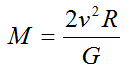# Viral Theorem

Theorem that relates the total kinetic energy of a self-gravitating body due to the motions of its constituent parts (T) to the gravitational potential energy (U) of the body.By re-arranging and making some simple assumptions about T and U for galaxies, this becomes:where M is the total mass of the galaxy, v is the mean velocity (combining the rotation and velocity dispersion) of stars in the galaxy, G is Newton’s gravitational constant, and R is the effective radius (size) of the galaxy. This equation relates two observable properties of galaxies (velocity dispersion and effective radius) to a fundamental, but unobservable, property – the mass of the galaxy. The comparison of mass estimates based on the virial theorem to estimates based on the luminosities provides one method of estimating the amount of dark matter in a galaxy or clusters of galaxies.